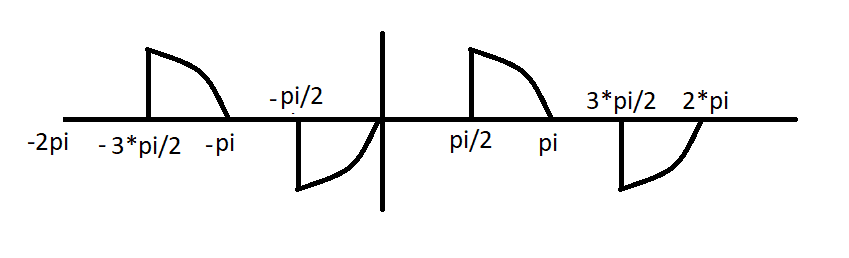# Fourier analysis & determination of Fourier Series

• Comp Sci
• Jason-Li
In summary, the function looks like it has an odd wave shape, but it is not odd because it has a 0 value at the center of the wave.

#### Jason-Li

Homework Statement
Sketch the waveform defined below and explain how you would obtain its fourier Series (I've attached the question. It's title is "wave.png"
Relevant Equations
As attached: It's title is "form.png"
ANY AND ALL HELP IS GREATLY APPRECIATEDI have found old posts for this question however after reading through them several times I am having a hard time knowing where to start.

I am happy with the sketch that the function is correctly drawn and is neither odd nor even. It's title is "wave1.png"
Ao=0 as the average value of the function is 0. I have proven this from working through Ao = 1/π ∫ f(x) dx with my limits of π/2 & π and 3π/2 & 2π.

Bn & An are where I am struggling, I have worked through and got answers but I don't think they are correct but can't see another way. So I did as follows with x = ωt. The limits of each integration are again π & π/2 and 2π & 3π/2.

An = 1/π ∫ f(x)cos(nx) dx
An = 1/π ∫ sin(x)cos(nx) dx + 1/π ∫ sin(x)cos(nx) dx
An = 1/π ∫ ((sin(x+nx))+sin(x-nx))/2+1/π ∫ ((sin(x+nx))+sin(x-nx))/2
An = 1/2π [ (-cos(x+nx))/(1+n) - (cos(x-nx))/(1-n) ] + 1/2π [ (-cos(x+nx))/(1+n) - (cos(x-nx))/(1-n) ]
An = 1/2π [[ (-cos(π+nπ))/(1+n) - (cos(π-nπ))/(1-n) ] - [ (-cos(π/2+n*π/2)/(1+n) - (cos(π/2-nπ/2))/(1-n) ] + 1/2π [[ (-cos(2π+n2π))/(1+n) - (cos(2π-n2π))/(1-n) ] - [ (-cos(3π/2+n*3π/2)/(1+n) - (cos(3π/2-n3π/2))/(1-n) ]
An = 1/2π [-(-cos(nπ))/(1+n) - (-cos(nπ))/(1-n)] + 1/2π [-(cos(n2π))/(1+n) - (cos(n2π))/(1-n)]
An = 1/2π [(2cos(nπ))/(1-n^2) + 1/2π [(2cos(n2π))/(1-n^2)]
An = (cos(πn)) / (π (1-n^2)) + (cos(2πn)) / (π (1-n^2))
An = (cos(πn)+(cos(2πn)) / (π (1-n^2)) This doesn't seem correct?

Other people have Bn working out to 0 however I can't figure out how to start at all, I had an initially thought that as it resembles an odd wave (but isn't odd due to the 0 values) that it would still only have sine terms?

Thanks in advance for any help, it really is appreciated!

#### Attachments

Last edited:
Does that describe the entire signal, or does it repeat? If it does repeat, then what will it look like from negative 2* pi to zero (in sections)

scottdave said:
Does that describe the entire signal, or does it repeat? If it does repeat, then what will it look like from negative 2* pi to zero (in sections)

I am assuming that it would repeat? There is no symmetry that I can see? It would be odd if there was no 'missing' parts of the wave however